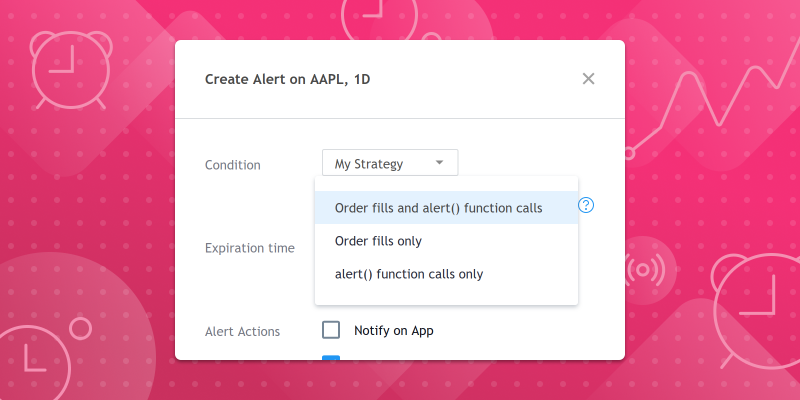January 25, 2021

1. 根據需要在腳本中包含盡可能多的`alert()`調用，並將每個調用都包含在定義觸發條件的`if` block中。
2. 使用圖表的“建立快訊”對話框為腳本建立一個快訊，然後選擇一種快訊類型，包括“ alert()函數調用”。

1) 每個K線上都會觸發腳本快訊，並且訊息中包含該K線的收盤價：

```//@version=4
study("Simple alert() example")
plot(close)
alert("Close = " + tostring(close), alert.freq_once_per_bar_close)```

2) 檢查三個指標的值：RSI、SMA、和動量。如果任何指標超過指定水平(或價格)，都會觸發腳本快訊，並且訊息中將包含指標名稱及其當前值。

```//@version=4
study("alert() with multiple indicators", overlay=true)
f_triggerSma()=>
_s  = sma(close, 14)
_co = crossover(close, _s)
_cu = crossunder(close, _s)
if _co
alert("Price (" + tostring(close) + ") crossing up SMA (" + tostring(_s) + ")", alert.freq_once_per_bar)
else if _cu
alert("Price (" + tostring(close) + ") crossing down SMA (" + tostring(_s) + ")", alert.freq_once_per_bar)
f_triggerRsi()=>
_r  = rsi(close, 7)
_co = crossover(_r, 70)
_cu = crossunder(_r, 30)
if _co
alert("RSI (" + tostring(_r) + ") crossing up 70 level", alert.freq_once_per_bar)
else if _cu
alert("RSI (" + tostring(_r) + ") crossing down 30 level", alert.freq_once_per_bar)
f_triggerMom()=>
_m  = mom(close, 14)
_co = crossover(_m, 0)
_cu = crossunder(_m, 0)
if _co
alert("Momentum (" + tostring(_m) + ")  crossing up 0 level", alert.freq_once_per_bar)
else if _cu
alert("Momentum (" + tostring(_m) + ")  crossing down 0 level", alert.freq_once_per_bar)

plot(sma(close, 14), "SMA")
f_triggerSma()
f_triggerRsi()
f_triggerMom()```

3) 在此示例中，檢查了一個RSI指標的值，但一次檢查了五個商品代碼。如果RSI交叉任何商品代碼的指定級別，將觸發腳本快訊，並且訊息將在交叉時包含商品和指標值。

```//@version=4
study("alert() with multiple symbols")
f_triggerRsi(_ticker)=>
_r = rsi(close, 7)
_x = crossover(_r,70)
_y = crossunder(_r,30)
[_co, _cu] = security(_ticker, timeframe.period, [_x, _y])
_msg = _ticker + ", " + timeframe.period + ": "
if _co
_msg := _msg + "RSI (" + tostring(_r) + ") crossing up 70 level"
alert(_msg,  alert.freq_once_per_bar_close)
else if _cu
_msg := _msg + "RSI (" + tostring(_r) + ")  crossing down 30 level"
alert(_msg,  alert.freq_once_per_bar_close)

plot(rsi(close, 7), "RSI", color=#8E1599)
band1 = hline(70, "Upper Band", color=#C0C0C0)
band0 = hline(30, "Lower Band", color=#C0C0C0)
fill(band1, band0, color=#9915FF, transp=90, title="Background")

f_triggerRsi(syminfo.tickerid)
f_triggerRsi("NASDAQ:MSFT")
f_triggerRsi("FX:EURUSD")
f_triggerRsi("NASDAQ:TSLA")
f_triggerRsi("NASDAQ:PYPL")```